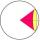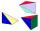# Frustum of a cone

A reservoir contains 28.54 m3 of water when completely full. The diameter of the upper base is 3.5 m while at the lower base is 2.5 m. Determine the height if the reservoir is in the form of a frustum of a right circular cone.

Result

h =  4.001 m

#### Solution:

$V = 28.54 \ m^3 \ \\ D_{ 1 } = 3.5 \ m \ \\ D_{ 2 } = 2.5 \ m \ \\ \ \\ r_{ 1 } = D_{ 1 }/2 = 3.5/2 = \dfrac{ 7 }{ 4 } = 1.75 \ m \ \\ r_{ 2 } = D_{ 2 }/2 = 2.5/2 = \dfrac{ 5 }{ 4 } = 1.25 \ m \ \\ \ \\ V = \dfrac{ 1 }{ 3 } \cdot \ \pi \cdot \ h ( r_{ 1 }^2 + r_{ 1 } \cdot \ r_{ 2 } + r_{ 2 }^2) \ \\ \ \\ h = \dfrac{ 3 \cdot \ V }{ \pi \cdot \ ( r_{ 1 }^2 + r_{ 1 } \cdot \ r_{ 2 } + r_{ 2 }^2) } = \dfrac{ 3 \cdot \ 28.54 }{ 3.1416 \cdot \ ( 1.75^2 + 1.75 \cdot \ 1.25 + 1.25^2) } \doteq 4.0005 = 4.001 \ \text { m }$

Leave us a comment of this math problem and its solution (i.e. if it is still somewhat unclear...):Be the first to comment!#### Following knowledge from mathematics are needed to solve this word math problem:

Looking for a statistical calculator? Tip: Our volume units converter will help you with the conversion of volume units.

## Next similar math problems:

1. SphereIntersect between plane and a sphere is a circle with a radius of 60 mm. Cone whose base is this circle and whose apex is at the center of the sphere has a height of 34 mm. Calculate the surface area and volume of a sphere.
2. Two ballsTwo balls, one 8cm in radius and the other 6cm in radius, are placed in a cylindrical plastic container 10cm in radius. Find the volume of water necessary to cover them.
3. Pine woodFrom a trunk of pine 6m long and 35 cm in diameter with a carved beam with a cross-section in the shape of a square so that the square had the greatest content area. Calculate the length of the sides of a square. Calculate the volume in cubic meters of lum
4. Surface of cubesPeter molded a cuboid 2 cm, 4cm, 9cm of plasticine. Then the plasticine split into two parts in a ratio 1:8. From each part made a cube. In what ratio are the surfaces of these cubes?
5. Body diagonalCalculate the volume of a cuboid whose body diagonal u is equal to 6.1 cm. Rectangular base has dimensions of 3.2 cm and 2.4 cm
6. Alien shipThe alien ship has the shape of a sphere with a radius of r = 3000m, and its crew needs the ship to carry the collected research material in a cuboid box with a square base. Determine the length of the base and (and height h) so that the box has the larges
7. Space diagonalThe space diagonal of a cube is 129.91 mm. Find the lateral area, surface area and the volume of the cube.
8. Cube in a sphereThe cube is inscribed in a sphere with volume 9067 cm3. Determine the length of the edges of a cube.
9. CubesOne cube is inscribed sphere and the other one described. Calculate difference of volumes of cubes, if the difference of surfaces in 257 mm2.
10. Prism XThe prism with the edges of the lengths x cm, 2x cm and 3x cm has volume 20250 cm3. What is the area of surface of the prism?
11. Cube in ballCube is inscribed into sphere of radius 241 cm. How many percent is the volume of cube of the volume of sphere?
12. Mystery of stereometrieTwo regular tetrahedrons have surfaces 88 cm2 and 198 cm2. In what ratio is their volumes? Write as a fraction and as a decimal rounded to 4 decimal places.
13. CuboidFind the cuboid that has the same surface area as the volume.
14. PyramidCuboid ABCDEFGH has dimensions AB 3 cm, BC 4 cm, CG 5 cm. Calculate the volume and surface area of a triangular pyramid ADEC.
15. Cube wallCalculate the cube's diagonal diagonal if you know that the surface of one wall is equal to 36 centimeters square. Please also calculate its volume.
16. Sphere areaA cube with edge 1 m long is circumscribed sphere (vertices of the cube lies on the surface of a sphere). Determine the surface area of the sphere.
17. Sphere vs cubeHow many % of the surface of a sphere of radius 12 cm is the surface of a cube inscribed in this sphere?